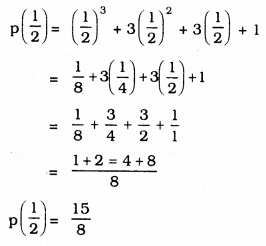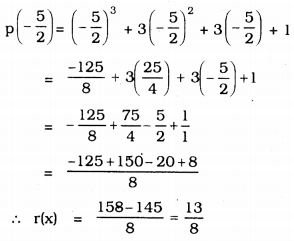# KSEEB Solutions for Class 9 Maths Chapter 4 Polynomials Ex 4.3

KSEEB Solutions for Class 9 Maths Chapter 4 Polynomials Ex 4.3 are part of KSEEB Solutions for Class 9 Maths. Here we have given Karnataka Board Class 9 Maths Chapter 4 Polynomials Exercise 4.3.

## Karnataka Board Class 9 Maths Chapter 4 Polynomials Ex 4.3

Question 1.
Find the remainder when x3 + 3x2 + 3x + 1 is divided by
i) x + 1
ii) $$x-\frac{1}{2}$$
iii) x
iv) x + π
v) 5 + 2x
i) p(x) = x3 + 3x2 + 3x + 1 g(x) = x – 1
Let x – 1 = 0, then
x = 1.
As per Remainder theorem, r(x) = p(x) = p(a)
p(x) = x3 + 3x2 + 3x + 1
p(1) = (1)3 + 3(1)2 + 3(1) + 1
= 1 + 3(1) + 3(1) + 1
= 1 + 3 + 3 + 1
P(1) = 8
∴ r(x) = p(x) = 8
∴ Remainder is 8.

ii) p(x) = x3 + 3x2 + 3x + 1 g(x) = $$x-\frac{1}{2}$$
If $$x-\frac{1}{2}=0$$ then $$x=\frac{1}{2}$$
p(x) = x3 + 3x2 + 3x + 1∴ r(x) = p(x) = p(a) = $$\frac{15}{8}$$
∴ Remainder is $$\frac{15}{8}$$

iii) p(x) = x3 + 3x2 + 3x + 1
g(x) = x
If x = 0, then
p(x) = x3 + 3x2 + 3x + 1
p(0) = (0)3 + 3(0)2 + 3(0) + 1
= 0 + 3(0) + 3(0) + 1
= 0 + 0 + 0 + 1
p(0) = 0
Remainder r(x) = 1.

iv) p(x) = x3 + 3x2 + 3x + 1
g(x) = x + π
If x + π = 0, then x = -π
p(x) = x3 + 3x2 + 3x + 1
p(-π) = (-π)3 + 3(-π)2 + 3(-π) + 1
p(-πt) = -π23 – 3π2 – 3π + 1
r(x) = -π3 – 3v2 – 3π+1

v) p(x) = x3 + 3x2 + 3x + 1
g(x) = 5 + 2x
If 5 + 2x = 0, then 2x = -5
$$x=-\frac{5}{2}$$
p(x) = x3 + 3x2 + 3x + 1Free handy Remainder Theorem Calculator tool displays the remainder of a difficult polynomial expression in no time.

Question 2.
Find the remainder when x3 – ax2 + 6x – a is divided by x – a.
p(x) = x3 – ax2 + 6x – a
If g(x) = x – a, then r(x) = ?
Let x – a = 0, then x = a
p(x) = x3 – ax2 + 6x – a
∴ p(a) = (a)3 – a(a)2 + 6(a) – a
= a3 – a3 + 6a – a
∴ p(a) = 5a
∴ r(x) = p(a) = 5a.

Question 3.
Check whether 7 + 3x is a factor of 3x3 + 7x.
$$x=-\frac{7}{3}$$Here, $$r(x)=-\frac{590}{9}$$. This is not equal to Zero.